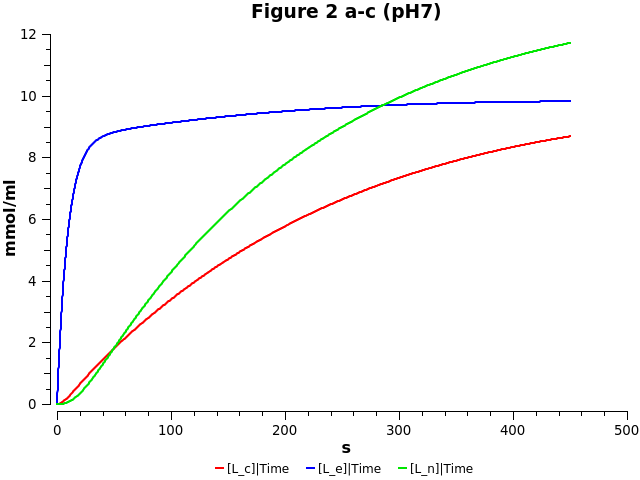## Evans2005 - Compartmental model for antineoplastic drug topotecan in breast cancer cellsModel Identifier
BIOMD0000000946
Short description

Compartment model for the antineoplastic drug topotecan. Modelling drug in its active lactone and inactive hydroxy acid forms in the medium, extracellular, cytoplasm and nucleus.

Format
SBML (L2V4)
Related Publication
• Compartmental modelling of the uptake kinetics of the anti-cancer agent topotecan in human breast cancer cells• Neil D. Evans, Rachel J. Errington, Michael J. Chapman, Paul J. Smith, Michael J. Chappell, Keith R. Godfrey
• Int. J. Adapt. Control Signal Process. , 1/ 2005 , Volume 19 , pages: 395-417 , DOI: 10.1002/acs.856
• School of Engineering, University of Warwick, Coventry CV4 7AL, U.K. Department of Medical Biochemistry, University of Wales College of Medicine, Cardiff CF14 4XN, U.K. School of MIS-Mathematics, Coventry University, Coventry CV1 5FB, U.K. Department of Pathology, University of Wales College of Medicine, Cardiff CF14 4XN, U.K
• A compartmental model for the in vitro uptake kinetics of the anti-cancer agent topotecan is proposed. This model provides a description of the activity of the drug, and subsequent delivery of active form to the nuclear DNA target. The unknown model parameters are estimated from two-photon laser-scanning microscopy data, which provide concentrations of topotecan (active plus inactive forms) in the extracellular region containing live human breast tumour cells (MCF-7 cell line), the cytoplasm and the nucleus. This determines an output structure for which the model is uniquely identifiable, that is, the unknown parameters are uniquely determined from noise-free, continuous and perfect data. The model allows in silico predictions of the dose dependence of target binding. Copyright 2005 John Wiley & Sons, Ltd.
Contributors
Submitter of the first revision: Matthew Roberts
Submitter of this revision: Krishna Kumar Tiwari
Modellers: Matthew Roberts, Krishna Kumar Tiwari

is (2 statements)
BioModels Database BIOMD0000000946
BioModels Database MODEL1805210001

hasTaxon (1 statement)
Taxonomy Homo sapiens

hasProperty (2 statements)
Mathematical Modelling Ontology Ordinary differential equation model
Ontology for Biomedical Investigations in vitro design

hasPart (2 statements)
isVersionOf (1 statement)
Pathway Ontology antineoplastic drug pathway

occursIn (1 statement)
Brenda Tissue Ontology MCF-7 cell

isDescribedBy (1 statement)

Curation status
Curated

Modelling approach(es)

Tags

#### Connected external resources

SBGN view in Newt Editor

Name Description Size Actions

### Model files

Evans2005.xml SBML L2V4 representation of Evans2005 - Compartmental model for antineoplastic drug topotecan in breast cancer cells 51.76 KB Preview | Download

Evans2005.cps Curated and annotated model COPASI file. Reproduced figure 2 a-c with parameters relating to pH7 condition. The assignment used for v1 was alpha*v0/(1+v0) which gives 0.36 and results in simulation results similar to publication results. Using the Ve/Vc assignment results in v1=3.6e4 and L_c and L_n behaviour is wrong. 64.11 KB Preview | Download
figure2_abc_pH7.png Reproduced figure 2 a,b,c for pH7. 15.38 KB Preview | Download
• Model originally submitted by : Matthew Roberts
• Submitted: May 21, 2018 10:43:13 AM
##### Revisions

(*) You might be seeing discontinuous revisions as only public revisions are displayed here. Any private revisionsof this model will only be shown to the submitter and their collaborators.

Legends
: Variable used inside SBML models

Species
Reactions
Reactions Rate Parameters
L_m = (-(k_om+k_mi))*L_m+k_cm*H_m+k_mo*v0*L_e (-(k_om+k_mi))*L_m+k_cm*H_m+k_mo*v0*L_e k_mi = 1.3974E-6; k_mo = 0.085551; k_om = 1.5599E-4; k_cm = 2.9553E-4; v0 = 1.5045E-5
H_e = (k_mi/v0*H_m+k_om*L_e)-(k_cm+k_mo)*H_e (k_mi/v0*H_m+k_om*L_e)-(k_cm+k_mo)*H_e k_mi = 1.3974E-6; k_mo = 0.085551; k_om = 1.5599E-4; v0 = 1.5045E-5; k_cm = 2.9553E-4
L_n = k_b/v2*(B_T-L_n)*L_c-(k_dl+k_dh)*L_n k_b/v2*(B_T-L_n)*L_c-(k_dl+k_dh)*L_n k_dl = 0.055522; v2 = 0.393244873341375; B_T = 89.972; k_dh = 1.5639E-7; k_b = 3.8085E-4
L_e = (k_mi/v0*L_m-(k_mo+k_om+k_i)*L_e)+k_cm*H_e+k_e/v1*L_c (k_mi/v0*L_m-(k_mo+k_om+k_i)*L_e)+k_cm*H_e+k_e/v1*L_c v1 = 0.362955164339553; k_i = 0.02211; k_mi = 1.3974E-6; k_mo = 0.085551; k_e = 0.0078915; k_om = 1.5599E-4; v0 = 1.5045E-5; k_cm = 2.9553E-4
L_c = ((k_i*v1*L_e-(k_e+k_oc)*L_c)+k_cc*H_c+k_dl*v2*L_n)-k_b*(B_T-L_n)*L_c ((k_i*v1*L_e-(k_e+k_oc)*L_c)+k_cc*H_c+k_dl*v2*L_n)-k_b*(B_T-L_n)*L_c v1 = 0.362955164339553; k_i = 0.02211; k_dl = 0.055522; v2 = 0.393244873341375; k_e = 0.0078915; k_oc = 1.2913E-4; B_T = 89.972; k_cc = 3.1578E-4; k_b = 3.8085E-4
H_m = (k_om*L_m-(k_cm+k_mi)*H_m)+k_mo*v0*H_e (k_om*L_m-(k_cm+k_mi)*H_m)+k_mo*v0*H_e k_mi = 1.3974E-6; k_mo = 0.085551; k_om = 1.5599E-4; k_cm = 2.9553E-4; v0 = 1.5045E-5
H_c = (k_oc*L_c-k_cc*H_c)+k_dh*v2*L_n (k_oc*L_c-k_cc*H_c)+k_dh*v2*L_n v2 = 0.393244873341375; k_oc = 1.2913E-4; k_dh = 1.5639E-7; k_cc = 3.1578E-4Curator's comment:
(added: 12 May 2020, 04:54:02, updated: 12 May 2020, 04:54:02)
Figure 2 a,b,c has been reproduced. Model encoded and results plotted using COPASI.